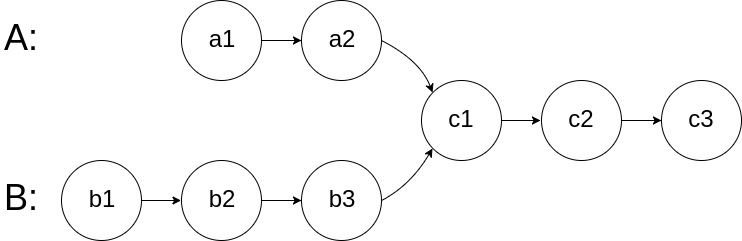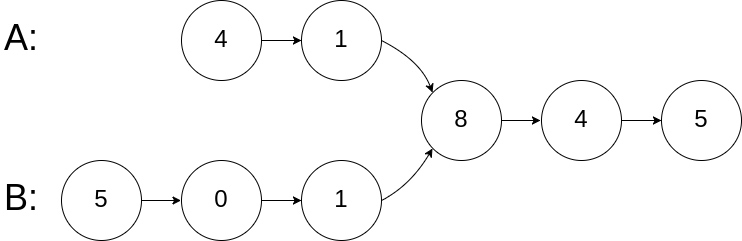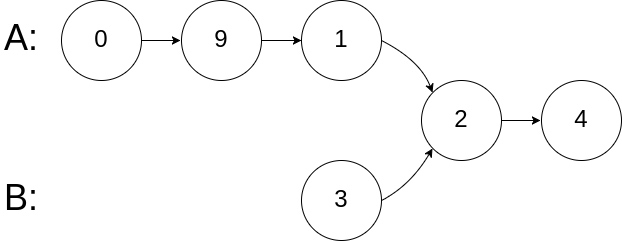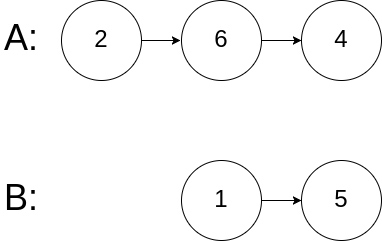posted in leetcode  链表  双指针

# 160. 相交链表

## 问题1. 如果两个链表没有交点，返回 null.
2. 在返回结果后，两个链表仍须保持原有的结构。
3. 可假定整个链表结构中没有循环。
4. 程序尽量满足 O(n) 时间复杂度，且仅用 O(1) 内存。

## 代码

### java

/**
* public class ListNode {
*     int val;
*     ListNode next;
*     ListNode(int x) {
*         val = x;
*         next = null;
*     }
* }
*/
public class Solution {
return null;
}
//首先遍历两个链表查询长度
int l1 = 0;
while (h1 != null) {
h1 = h1.next;
l1++;
}
int l2 = 0;
while (h2 != null) {
h2 = h2.next;
l2++;
}

//长度更长的那个需要先走几步，直到两个长度相等时才同时前进。
int dif = l1-l2;
if (dif > 0) {
while(dif-- >0) {
h1 = h1.next;
}
} else if (dif < 0) {
while(dif++ < 0) {
h2 = h2.next;
}
}
int len = Math.min(l1, l2);
while (h1 != h2){
h1 = h1.next;
h2 = h2.next;
}
return h1;
}
}